Linear Scale

Given a scale diagram we can make drawing measurements.

Similarly, real (actual) measurements can be made on a real object.

Linear scale shows, the relationship between drawing (scale) length and actual length.

Linear scale of a diagram is given in statement or ratio form.

When the ratio form is used the unit of measurement for the drawing length and actual length is the same.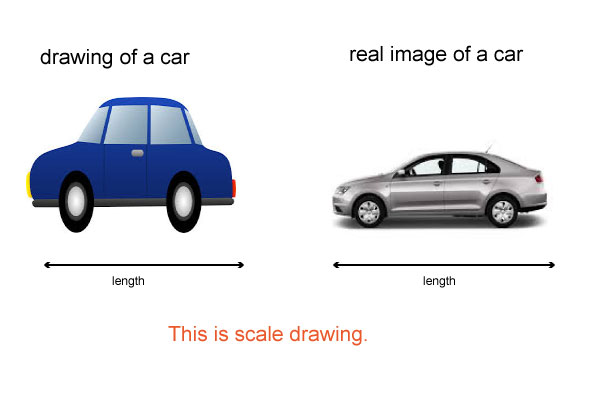It is not always possible to draw on paper the actual size of real-life objects

such as the real size of a car, an airplane, house

we need scale drawings to represent the size like the one you have seen.

#### Example 1

In a scale diagram 1cm represents 50 m

(a) Write the scale in ratio form.

(b) Find the drawing length for 1.25 km.

(c) Find the actual length in kilometers corresponding to a length of 10.5cm on the diagram.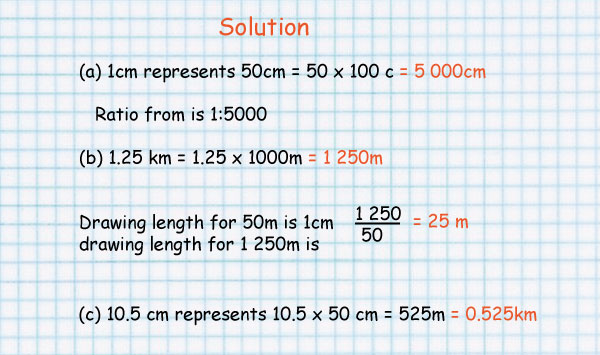#### Example  2

In a diagram of a plan of a factory a length of 2.5 cm represents an actual length of 12.5 m

(a) Work out the linear scale in ratio form.

(b) Find the distance on the plan between two buildings which are 35 m apart.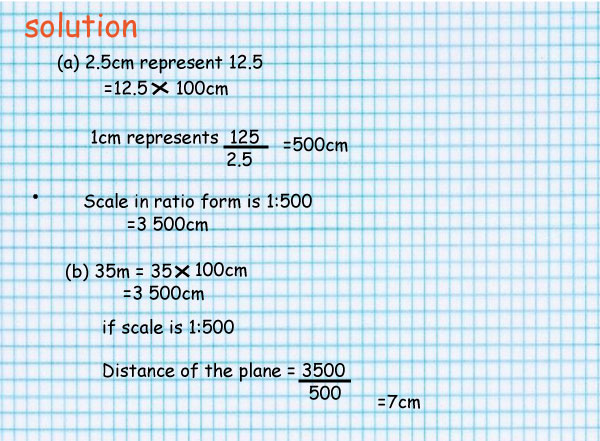## Conversions to recall

• 1 km =1000 m
• 1 km = 100,000 cm
• 1 m = 100 cm
• 1 ha = 10,000 m2

## Scale in statement form

1cm represents 3m can be written as: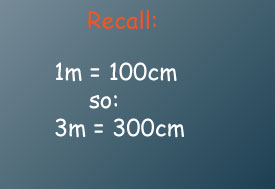1cm represents 300cm.

It can also be written as a ratio

1:300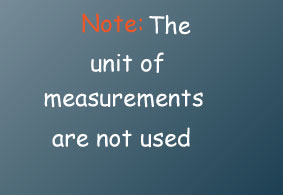Read as; one to three hundred.

For every unit of drawing there are three hundred similar units of real length.

Drawing length: real length

#### Write each of the following scales as a ratio

(i)1cm represents 4km
(ii)1cm represents 5m
(iii)1cm represents 200m

#### Complete the following statements

(i)The scale 1:10000 can be written as 1cm represents____metres.
(ii)The scale 1:4000000 can be written as 1cm represents ____km.

#### Solution

(i)1:400000    (ii)1:500   (iii)1:20000  (iv)100m   (v)40km

### Why are scale drawings important?

Careers that involve construction, architecture, city planning, design and map-reading all require knowledge of scale drawings

You also need to able to use scale-drawings when you are travelling (maps).

#### Example

use the map of Maine to estimate the distance of China and New Sweden.